### The Remainder Theorem

#### Rule to remember:

• If a polynomial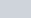is divided by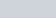then the remainder is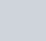.

In the video tutorial I demonstrate this.

The Remainder Theorem Rule to remember: If a polynomial $f(x)$ is divided by $(ax-b)$ then the remainder is ${f \left( \dfrac{b}{a} \right )}$. In the video tutorial I demonstrate this. Finding the remainder when a cubic polynomial is divided by x+1 In the vide

## Finding the remainder when a cubic polynomial is divided by x+1

In the videos that follow, I run through some typical remainder theorem questions that you are likely to encounter. I start with this example.

#### Example in the video

1. Find the remainder when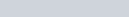is divided by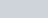The Remainder Theorem Rule to remember: If a polynomial $f(x)$ is divided by $(ax-b)$ then the remainder is ${f \left( \dfrac{b}{a} \right )}$. In the video tutorial I demonstrate this. Finding the remainder when a cubic polynomial is divided by x+1 In the vide

## Finding the remainder when a quartic polynomial is divided by 2x-1

#### Example in the video

1. Find the remainder whenis divided by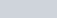The Remainder Theorem Rule to remember: If a polynomial $f(x)$ is divided by $(ax-b)$ then the remainder is ${f \left( \dfrac{b}{a} \right )}$. In the video tutorial I demonstrate this. Finding the remainder when a cubic polynomial is divided by x+1 In the vide

## Finding constants in a polynomial given the remainders

#### Example in the video

1. When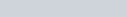is divided bythe remainder is 3 and when divided bythe remainder is -27. Find a and b.
The Remainder Theorem Rule to remember: If a polynomial $f(x)$ is divided by $(ax-b)$ then the remainder is ${f \left( \dfrac{b}{a} \right )}$. In the video tutorial I demonstrate this. Finding the remainder when a cubic polynomial is divided by x+1 In the vide